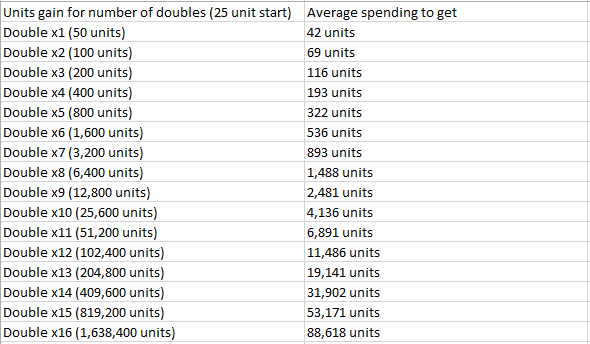# Explaining double or nothing

Ok so I was playing double or nothing and I noticed that it wasn’t actually a 50% chance to double, but felt like it was a bit more in the players favor, so I made a tally of when I get a double and when I get nothing and here is what I got after 1200 tries:

Double: 707
Nothing: 493

It calculates to a 59% chance that a player doubles and 41% that a player gets nothing, so it’s likely that the devs decided on a 60% chance that you would double. Now what would be the strategy to earn the most amount of money in this case? Here is a formula I wrote that would express the net gain:

Net gain = un*(2^x)*(p^x) - un

where u=units spent on an attempt n=number of attempts p=probability of a double x=number of doubles per attempt

We already estimated p=0.6 and let u and n = 1 to simplify the equation you get:

Net gain = 1.2^x - 1

From this you can see that the more you double per attempt, the higher the gain, so it is always in your advantage to double.

I attached a picture of table showing how much you would have to spend (on average) to get different double multipliers and how much you would gain. For example, if you spend more than 88,618 units on double or nothing (without cashing out), there is more than a 50% chance that you would get a x16 Double (1,638,400 units)## EDIT

I would just like to point out that I made the assumption that the probability of getting a double is constant. This is likely not be true. I did notice that getting a double gets harder as you double more. I tallied the number of doubles I got for each double multiplier, but getting the data for higher doubles is difficult and time consuming and I do need more data to be confident. Here is what I got:

Attempts 1000 | Net units if I cash out here each attempt
x1 Double 585 | 4250 Units
x2 Double 339 | 8900 Units
x3 Double 182 | 11400 Units
x4 Double 104 | 16600 Units
x5 Double 54 | 18200 Units
x6 Double 33 | 27800 Units
x7 Double 13 | 16600 Units
x8 Double 8 | 26200 Units
x9 Double 4 | 26200 Units
x10 Double 0 | -25000 Units

Notice I don’t have much data for the higher doubles, so it’s not accurate for higher doubles, but you can see that the net units increase until a certain point (may be around x6?). It makes sense why the devs would make probability of a double lower for higher doubles, because it can be abused to get a ridiculous amount of money.

8 Likes

lmao
i thought it would be losing in general, i used to play this and lost more than 30k units but not even x14.

2 Likes

Epic.

You’re way overthinking this. Just because u got double 59% of the time in that session, doesn’t mean it’s not a 50% chance to double. Just means you got lucky.

If you flip a coin, you have a 50% chance of getting heads. If you flip the coin 10 times, you aren’t guaranteed to get 5 heads and 5 tails. You could get really lucky and get 10 heads. That doesn’t mean flipping a coin has 100% chance to land on heads.

Yeah, I doubt that the odds are anything but 50/50. The devs previously described Double or Nothing as “pure, concentrated gambling”

I did 1200 attempts and got significantly more double than nothing. I then did 1000 attempts and still got significantly more double than nothing. We can calculate the p value to determine if it is in fact significant.

Lets test the null hypothesis that it’s always a 50% chance to double:

Expected double:nothing is 1:1

Total: 1200

observed doubles = 707
expected doubles = 600
observed nothing = 493
expected nothing = 600

chi square = (707-600)^2/600 + (493-600)^2/600 = 38.2
There is one degree of freedom

Using the online p value calculator, the p value < 0.00001

We reject the null hypothesis

So its extremely unlikely that it is 50%. You can try it yourself. Go to the double or nothing machine double once and cash out. Do that over and over again and you will find that you’re getting significantly more doubles than nothing.

2 Likes

i think having it above the RTP is intentional to get people bet further.

Considering I have no earthly idea what you just said, I’m just gonna assume you know what you’re talking about and agree with you. Lol

the question is also how much time you are willing to spend.
(double or nothing part)